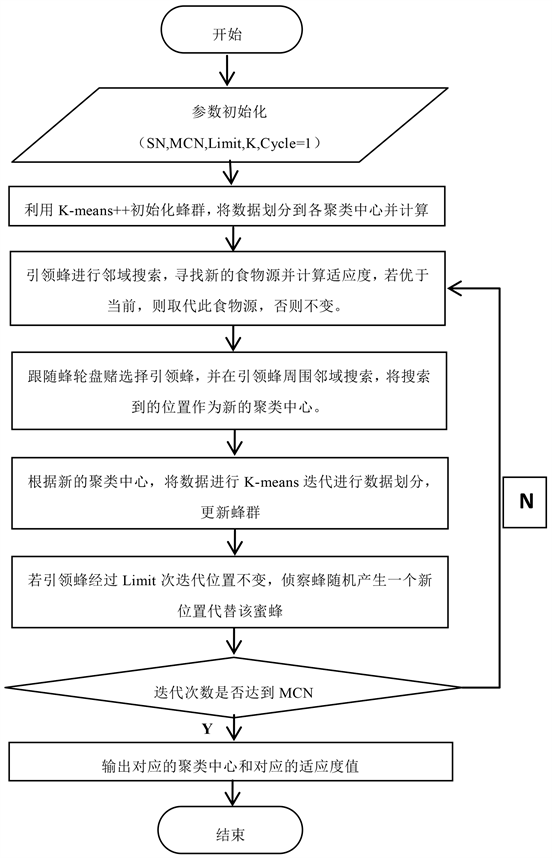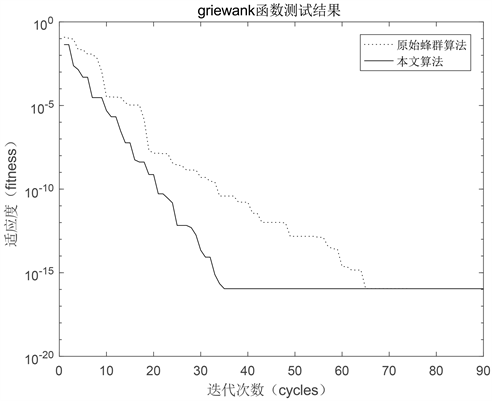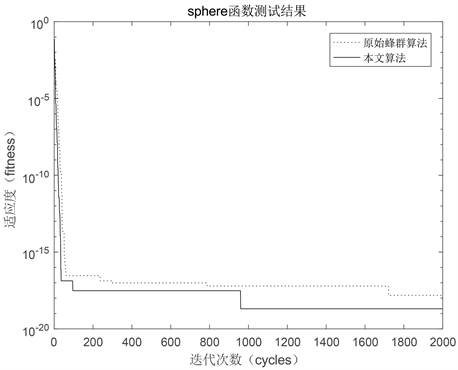# 基于人工蜂群与K-Means的改进混合聚类算法Improved Hybrid Clustering Algorithm Based on Artificial Bee Colony Algorithm and K-Means Algorithm

DOI: 10.12677/AIRR.2020.92011, PDF, HTML, XML, 下载: 104  浏览: 673  国家自然科学基金支持

Abstract: In order to overcome the disadvantages of K-Means clustering algorithm, such as over dependence on the initial clustering center, easily falling into local optimum, and the premature and slow con-vergence of the artificial bee colony algorithm due to the limitations of search strategies, a hybrid clustering method combining the improved global artificial bee colony algorithm and K-Means++ algorithm is proposed, which makes full use of the characteristics of the improved global artificial bee colony algorithm and K-Means++ algorithm. It can optimize the location of the initial clustering center and the convergence speed is fast. By combining the two, K-Means can search globally and jump out of the local optimal solution. The experiments are carried out with the Wine data set and balance-Scale data set in the UCI database. The results show that the improved global artificial bee colony algorithm has faster convergence speed and better optimization effect than the standard artificial bee colony algorithm. Compared with the original K-Means algorithm, the hybrid clustering algorithm proposed in this paper has better stability, fewer iterations, faster conver-gence speed and better clustering effect.

1. 引言

2. 相关算法简介

2.1. 原始K-Means算法

Step 1：随机选取k个簇中心点；

Step 2：遍历所有数据，将每个数据划分到欧式距离最近的中心点中；

Step 3：计算每个聚类的平均值，并作为新的簇中心点；

Step 4：重复2~3，直到某个中止条件。

2.2. 人工蜂群算法

Step1：初始化蜂群数NP、食物源个数SN，最大迭代次数MCN、食物源停滞的最大次数Limit和确定搜索空间D，通常有SN = NP/2；在设置的区间内按照公式(1)随机生成SN个食物源并根据公式(2)计算其适应度值fitness。

${X}_{ij}={b}_{j}+rand\left(0,1\right)\left({a}_{i}-{b}_{j}\right)$ ; $\left(i=1,2,3,\cdots ,\text{SN}\right)$ (1)

$fitness=\left\{\begin{array}{l}\frac{1}{1+fitness}\\ 1+abs\left(fitness\right)\end{array}$ (2)

Step2：引领蜂由式(3)进行邻域搜索，产生一个候选食物源并计算fitness，

${V}_{ij}={x}_{ij}+{R}_{ij}\left({x}_{m}{}_{j}-{x}_{kj}\right)$ (3)

Step3：跟随蜂按照轮盘赌选择引领蜂，根据公式(3)更新当前位置，fitness较大，被更新的可能越大，选择概率为公式(4)：

${P}_{i}=fi{t}_{i}/\underset{i=1}{\overset{SN}{\sum }}fi{t}_{i}$ ; $i=1,2,3,\cdots ,\text{SN}$ (4)

Step4：经过Limit次循环后某个解没有被更新，则放弃当前食物源，此引领蜂转成侦查蜂。

Step5：完成MCN次迭代后，输出fitmax的最优解。

3. 一种基于人工蜂群与K-Means的改进混合聚类算法(IGABC-K-Means++)

3.1. K-Means++算法

Step 1：随机寻找一个点作为中心点；

Step 2：计算其他点到目前的全部簇中心点的距离(最开始只有一个中心点)；

Step 3：利用公式(5)计算出映射到对应点的概率。

${P}_{i}=\frac{D{\left(k\right)}^{2}}{\underset{i=0}{\overset{m}{\sum }}D{\left(i\right)}^{2}}$ (5)

Step 4：根据Step 3中计算出的概率利用轮盘法随机选择出一个中心点，然后重复步骤2，3，4，直至找到全部中心点。

3.2. 适应度函数设计

$fitnes{s}_{i}=\underset{j=1}{\overset{k}{\sum }}\underset{{x}_{i}\in {c}_{j}}{\sum }d\left({x}_{i},Cente{r}_{j}\right)/C{N}_{j}$ (6)

$\underset{j=1}{\overset{k}{\sum }}\underset{{x}_{i}\in {c}_{j}}{\sum }d\left({x}_{i},Cente{r}_{j}\right)$ 表示第j类的类内距。CNj表示属于第j类的样本数。

3.3. GABC算法

${V}_{ij}={x}_{ij}+{R}_{ij}\left({x}_{m}{}_{j}-{x}_{kj}\right)+\phi \left({x}_{best}-{x}_{ij}\right)$ (7)

Rij$\left[-\text{1},\text{1}\right]$ 中的一个随机数，xbest表示全局最优食物源， $\phi$$\left[0,C\right]$ (C是一个正数)中的一个随机数，经多次实验，当 $C=1.5$ 时效果最好。

3.4. 贪婪选择

${P}_{i}=1-\frac{fi{t}_{i}}{\underset{i=1}{\overset{SN}{\sum }}fi{t}_{i}}$, $i=1,2,3,\cdots ,\text{SN}$ (8)

3.5. IGABC-K-Means++算法的实现

Step 1：模型开始，设置引领蜂、跟随蜂的数量(一般情况下，二者相等)；最大迭代次数MCN及控制参数Limit；聚类簇数K，Cycle = 1；利用K-Means++初始化SN个蜂群。

Step 2：对初始蜂群进行聚类划分，根据公式(6)计算每只蜜蜂的fitness，将值较小的50%为引领蜂，值较大的50%为跟随蜂。

Step 3：引领蜂利用式(7)进行邻域搜索，得到新位置，对比两个位置的fitness，按照贪婪选择原则，如果fit < fit，则新位置取代原位置；否则，保持原位置。并且将食物源的停止次数加1；当所有引领蜂完成邻域搜索后，根据式(8)计算概率Pi。

Step 4：跟随蜂利用Pi并基于轮盘赌原则选择引领蜂。当跟随蜂完成选择后，利用式(7)对邻域进行搜索，同样按照贪婪选择原则选择fiti小的位置。

Step 5：跟随蜂搜索结束，用K-Means对得到的位置进行聚类划分，更新蜂群。

Step 6：如果某引领蜂在Limit次迭代后，结果都没有改变，则变为侦察蜂，并随机产生一个新食物源。

Step 7：如果当前迭代次数达到最大次数MCN转向步骤8，否则转向步骤3，Cycle = Cycle + 1。

Step 8：输出聚类中心和对应的fitness，算法结束。算法流程图如图1所示：Figure 1. IGABC-K-Means++ algorithm flowchart

4. 实验结果及分析

4.1. IGABC算法性能测试

Griewank函数：

$f\left(x\right)=\frac{1}{4000}\underset{i=1}{\overset{D}{\sum }}{X}_{i}^{2}-\underset{i=1}{\overset{D}{\prod }}\mathrm{cos}\left(\frac{{x}_{i}}{\sqrt{i}}\right)+1$ ${\left[-600,600\right]}^{D}$

Sphere函数：

$f\left(x\right)=\underset{i}{\overset{D}{\sum }}{x}_{i}^{2}$ ${\left[-100,100\right]}^{D}$

4.2. IGABC-K-Means++算法性能测试Figure 2. Fitness change of different algorithms in the Griewank functionFigure 3. Fitness change of different algorithms in sphere functionTable 1. Data sets used in the experimentTable 2. Clustering comparison results of wine dataTable 3. Cluster comparison results of Balance-Scale data

ABC+K-Means算法是把原始的ABC算法与传统的算法融合在一起，想比较而言，此算法的寻优能力比传统K-Means算法好一些，标准差减小，但ABC算法易早熟的问题仍然存在，所以算法后期收敛速度缓慢，需要消耗的时间更长；

IABC-K-Means算法是文献  提出的算法，采用最大最小距离积法产生初始聚类中心，克服了传统K-Means鲁棒性较差的缺点，聚类效果得到改善；

IGABC-K-Means++算法在选择的初始点时可以更好的反映数据实际分布，既保证了聚类准确度和算法效率，而且算法表现出很好的稳定性。

5. 结论

NOTES

*通讯作者。

  廖伍代, 朱范炳, 王海泉, 孙雪凯. 基于人工蜂群优化的Ｋ均值聚类算法[J]. 计算机测量与控制, 2018, 26(4): 136-138.  杨俊闯, 赵超. K-Means聚类算法研究综述[EB/OL]. 计算机工程与应用(网络首发论文). http://kns.cnki.net/kcms/detail/11.2127.TP.20191015.1136.006.html, 2019-10-15.  刘薇, 刘伯嵩, 王洋洋. 基于改进鱼群和K-Means的混合聚类算法[J]. 计算机工程与应用, 2013, 49(22): 119-122.  喻金平, 张勇, 廖列法, 等. 一种改进的混合蛙跳和k均值结合的聚类算法[J]. 计算机工程与科学, 2016, 38(2): 356-362.  熊众望, 罗可. 基于改进的简化粒子群聚类算法[J]. 计算机应用与研究, 2014, 31(12): 3550-3552.  邓海, 覃华, 孙欣. 一种优化初始中心的K-Means聚类算法[J]. 计算机技术与发展, 2013, 23(11): 42-45.  Lu, B. and Ju, F. (2012) An Optimize Genetic K-Means Clustering Algorithm. CSIP 2012: Proceedings of the 2012 International Con-ference on Computer Science and Information Processing, 2012, 1296-1299.  刘三阳, 张平, 朱明敏. 基于局部搜索的人工蜂群算法[J]. 控制与决策, 2014, 29(1): 123-128.  葛宇, 梁静, 王学平. 基于极值优化策略的改进的人工蜂群算法[J]. 计算机科学, 2013, 40(6): 247-251.  周长喜, 毛力, 吴滨, 等. 基于当前最优解的人工蜂群算法[J]. 计算机工程, 2015, 41(6): 147-151.  毕晓君, 宫汝江. 一种结合人工蜂群和k-均值的混合聚类算法[J]. 计算机应用研究, 2012, 29(6): 2040-2042.  喻金平, 郑杰, 梅宏标. 基于改进人工蜂群算法的k均值聚类算法[J]. 计算机应用, 2014, 34(4): 1065-1069.  Zhu, G.P. and Kwong, S. (2010) Gbest-Guided Artificial Bee Colony Algorithm for Numerical Function Optimization. Applied Mathematics and Computation, 27, 1341-1348.  罗可, 易斌. 一种基于改进蜂群的K-Means聚类算法[J]. 长沙理大学学报(自然科学版), 2016, 13(4): 85-89.  常扣扣. 基于改进人工蜂群算法的K-Means聚类算法[D]: [硕士学位论文]. 兰州: 兰州交通大学, 2017.